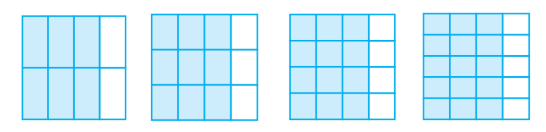## Filters

Sort by :
Q

Capacitance in a medium

C'=KC

K=C'/ C=110/50=2.2

A man of mass () is sitting in a train movingwith velocity. He observe that a block of massis moving towards him with velocity. Then the kinetic energy of block w.r.t  man is (Assume)

K.E of the block from the reference frame of the train is

A block of mass m is kept on a platform which starts from rest with constant acceleration g/2 upward, as shown in the figure. Work done by normal reaction on block in time t is:By balancing the forces in vertical direction, we get: and displacement is given by equation: where u=0 and a=g/2 and we know,

When the rms voltages VL, VC and VR are  measured respectively across the inductor L, the capacitor C and the resistor R in a series  LCR  circuit  connected  to  an AC source, it is found that the ratio VL : VC : VR = 1 : 2 : 3. If the rms voltage of the AC source is 100 V, then VR is close to ?

E=CV2

where

V=Ed

Substitute the value of C and V in the first equation

Write these in ascending and also in descending order.

(a)

(b)

(c)

option A

Which is the larger fraction?

(i)   or

(ii)   or

(iii)   or

The fractions are shown below using greater than or less than sign                      (i)                           (ii)                         (iii)

You get one-fifth of a bottle of juice and your sister gets one-third of the same size of a bottle of juice. Who gets more?

one third is greater potion than one fifth

Is in its simplest form?

Yes,  is in its simplest form because 49 and 64 has no common divisor.

Write the simplest form of :

(i)

(ii)

(iii)

(iv)

(v)

A

Find five equivalent fractions of each of the following:

(i)

(ii)

(iii)

(iv)

(i)                 (ii)                 (iii)                 (iv)

Identify the fractions in each. Are these fractions equivalent?(i)                                       (ii)                          (iii)                              (iv)  all fractions in it simplest form is   So all fractions are equivalent

Give example of four equivalent fractions.

Four example of  equivalent fractions are :

Are and ;  and   ;  and  and equivalent? Give reason

and ;  and    are not equivalent because    and    but

Fill up using one of these: ‘’, ‘’ or ‘

(a)

(b)

(c)

(d)

(e)

(a)        (b)          (c)       (d)  (e)

A fraction is given. How will you decide, by just looking at it, whether, the fraction is

(a) less than ?

(b) equal to ?

(a) If the numerator is smaller than the denominator, then the fraction will be less than . (b) If the numerator is equal to the denominator, then the fraction will be equal to .

Give a proper fraction :

(a) whose numerator is  and denominator is
(b) whose denominator is and numerator is

(c) whose numerator and denominator add up to . How many fractions of this kind can you make?

(d) whose denominator is more than the numerator.

(Give any five. How many more can you make?)

A proper fraction whose: (a) numerator is  and denominator is  =                                                                                                                                        (b) denominator is and numerator is   =                       (c) numerator and denominator add up to .  Pairs of numbers having sum 10 =  Therefore, the proper fractions are  (d) denominator is ...

How many fractions lie between  and ? Think, discuss and write your answer?

There are infinite number of fractions between  and . A fraction is of form  and for a number lying between  and , the numerator has to be less than the denominator.
Exams
Articles
Questions